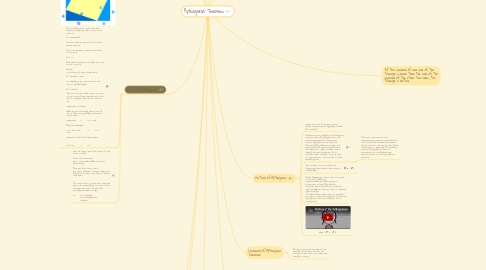# Pythagoras’ TheoremTOH YEE SHUEN, KAELA 2S
Get Started. It's FreePythagoras’ Theorem## 4. History of Pythagoras

### 4.2. B.C.Ancient clay tablets from Babylonia indicate that the Babylonians in the second millennium B.C., 1000 years before Pythagoras, had rules for generating Pythagorean triples, and understood the relationship between the sides of a right triangle, and, in solving for the hypotenuse of an isosceles right triangle, came up with an approximation of accurate to five decimal places.

4.2.1. A Chinese astronomical and mathematical treatise called Chou Pei Suan Ching (The Arithmetical Classic of the Gnomon and the Circular Paths of Heaven, ca. 500-200 B.C.), possibly predating Pythagoras, gives a statement of and geometrical demonstration of the Pythagorean theorem.

## 10. Trigo Ratios

### 10.1. Conditions

10.1.1. Trigo Ratios can only be used on RIGHT ANGLED triangles

### 10.2. Identifying Lengths

10.2.1. The Opposite length (Opp) is the length opposite the angle you are finding

10.2.2. The Hypotenuse (Hyp) is the longest side of the right angled triangle

10.2.3. The Adjacent length is the length next to the angle you are finding

10.2.4. eg. angle BAC: opp-a, hyp-c, adj-b

### 10.3. Finding Trigo ratios

10.3.3. SOH : sin-1 (opp/hyp)

### 10.4. Application

10.4.1. used for finding the height of buildings, mountains, etc.

10.4.2. used in navigation, to find the distance of the shore from a point in the sea

10.4.3. used in oceanography to find the height of the tides in the sea

10.4.4. used in finding the distance between celestial bodies

10.4.5. Architects use trigonometry to calculate structural load, roof slopes, ground surfaces, etc.

## 11. Toa Cah Soh

### 11.1. Toa

11.1.1. When finding an angle: 1. take the opposite over the adjacent and equate it to the angle that you want to find multiplied by the tangent sign (note: tangent angle can NEVER be a right angle) 2. throw the tangent over the equal gin and multiply (opp/adj) by the negative tangent 3. punch it in the calculator to get the angle

11.1.2. When finding the length of a side: 1. tangent angle = opp/given adj or given opp/adj 2. if finding for opp, take the tangent angle multiplied by the given adj/ if finding adj, take the given opp and divide it by the tangent angle

### 11.2. Cah

11.2.1. When finding an angle: 1. cosine the angle you are looking for = given adj/given hypo 2. throw the cosine over the equal sign 3. multiple adj/hypo by negative cosine

11.2.2. When finding length: 1. cosine given angle = adj/given hypo or given adj/hypo 2a. finding adj: multiply cosine angle by given hypo 2b. finding hypo: divide cosine angle by given adj

### 11.3. Soh

11.3.1. When finding an angle: 1. sine the angle you are looking for = given adj/given hypo 2. throw the sine over the equal sign 3. multiple adj/hypo by negative sine

11.3.2. When finding length: 1. sine given angle = opp/given hypo or given opp/hypo 2a. finding opp: multiply sine angle by given hypo 2b. finding hypo: divide sine angle by given opp

## 12. Results from Clinometer Task

### 12.2. Lamp outside Sports Hall

12.2.1. 49 degrees

12.2.2. 8 kerifeet

12.2.3. height of lamppost = tan(49) (8) = 9.20 kf (kerifeet) = 2.02m

### 12.3. small netball hoop

12.3.1. 33 degrees

12.3.2. 7 kerifeet

12.3.3. Height of small netball hoop = tan (33) (7) = 4.55kf = 1.00m

### 12.4. fan in Sports hall

12.4.1. 26 degrees

12.4.2. 10 kerifeet

12.4.3. Height of fan = tan (26) (10) = 4.88 kf = 1.07m

### 12.5. timing/score board in Sports hall

12.5.1. 41.5 degrees

12.5.2. 17-18 kerifeet

12.5.3. Height of scoreboard = tan (41.5) (17.5) = 15.48kf = 3.41m